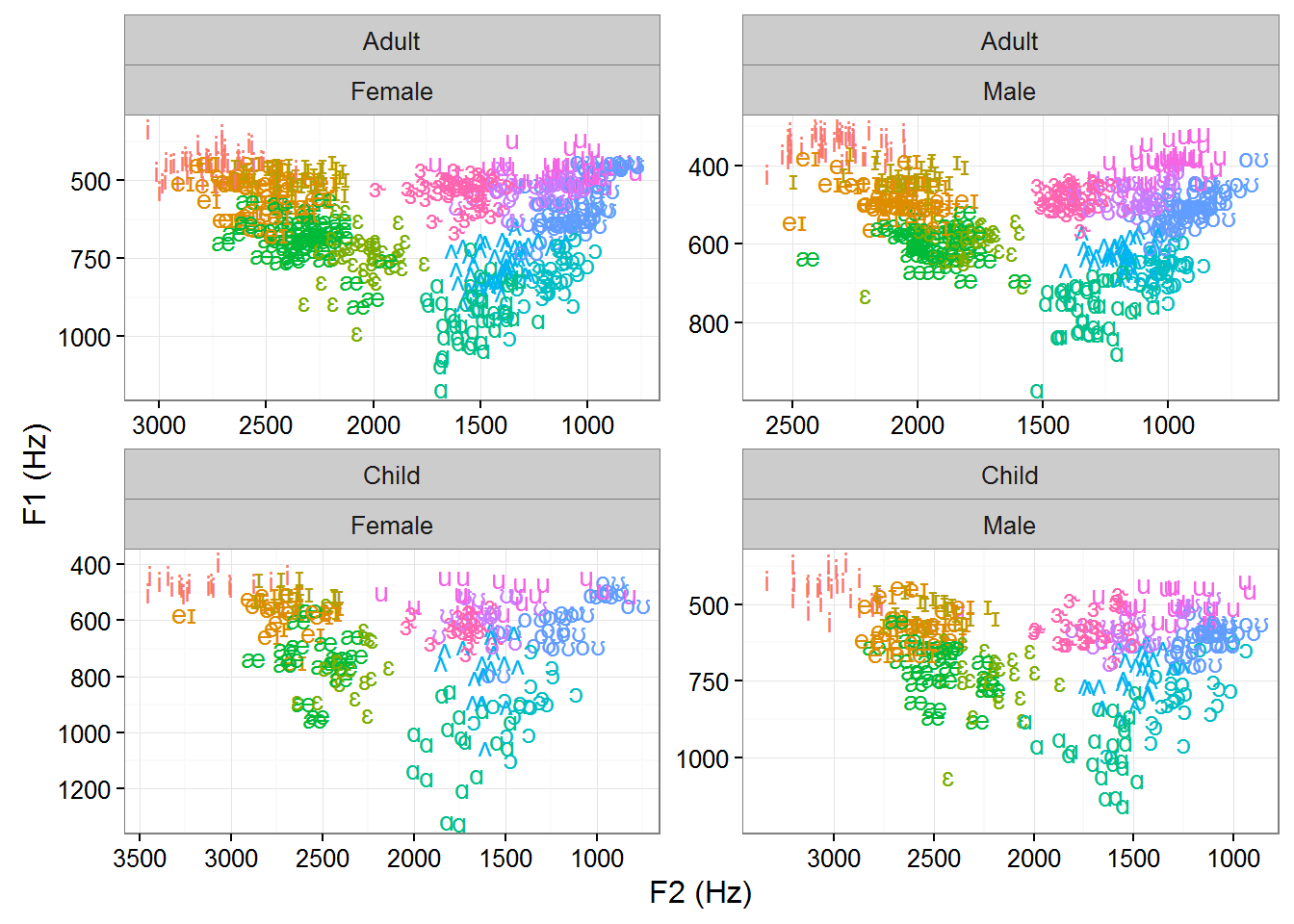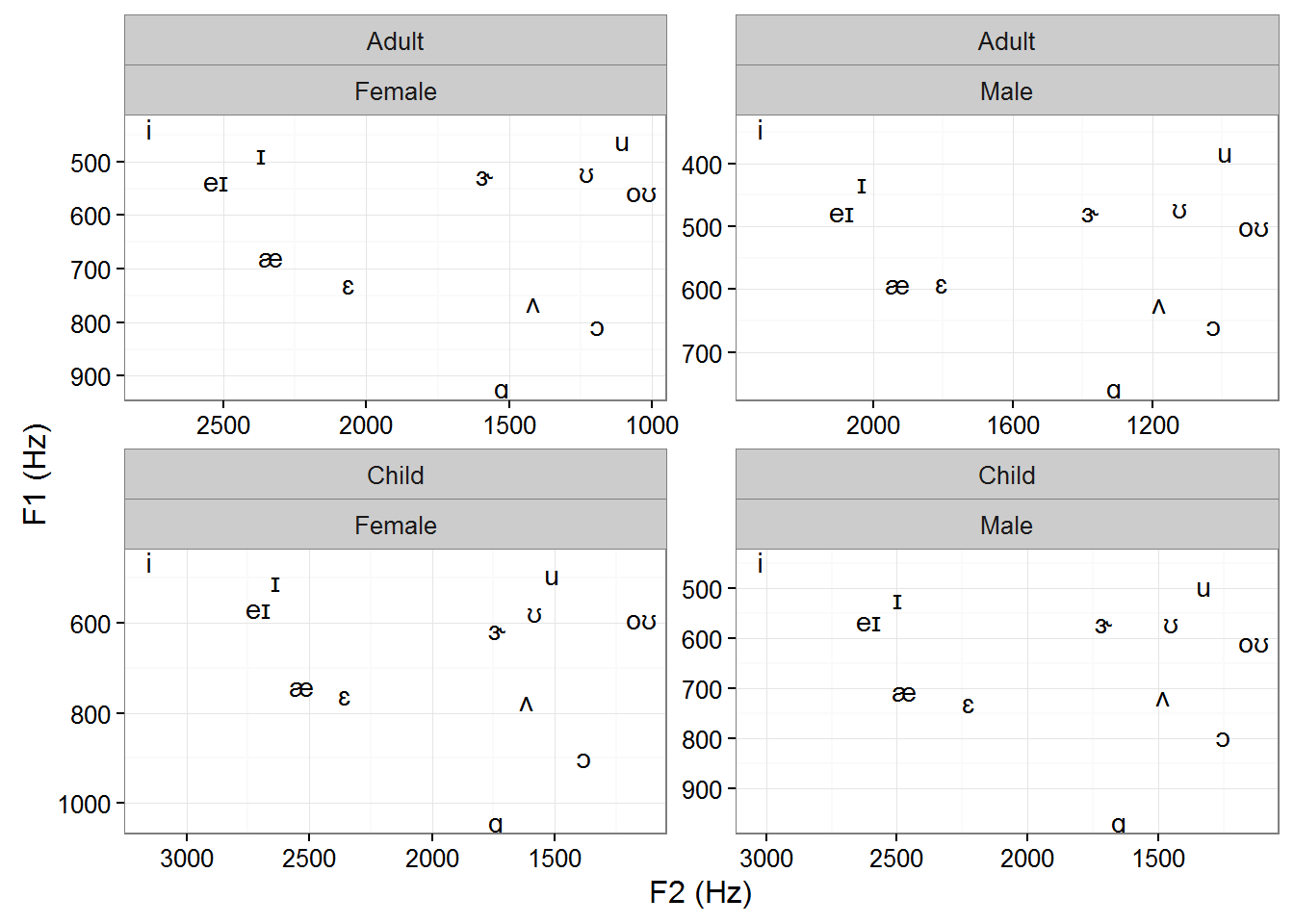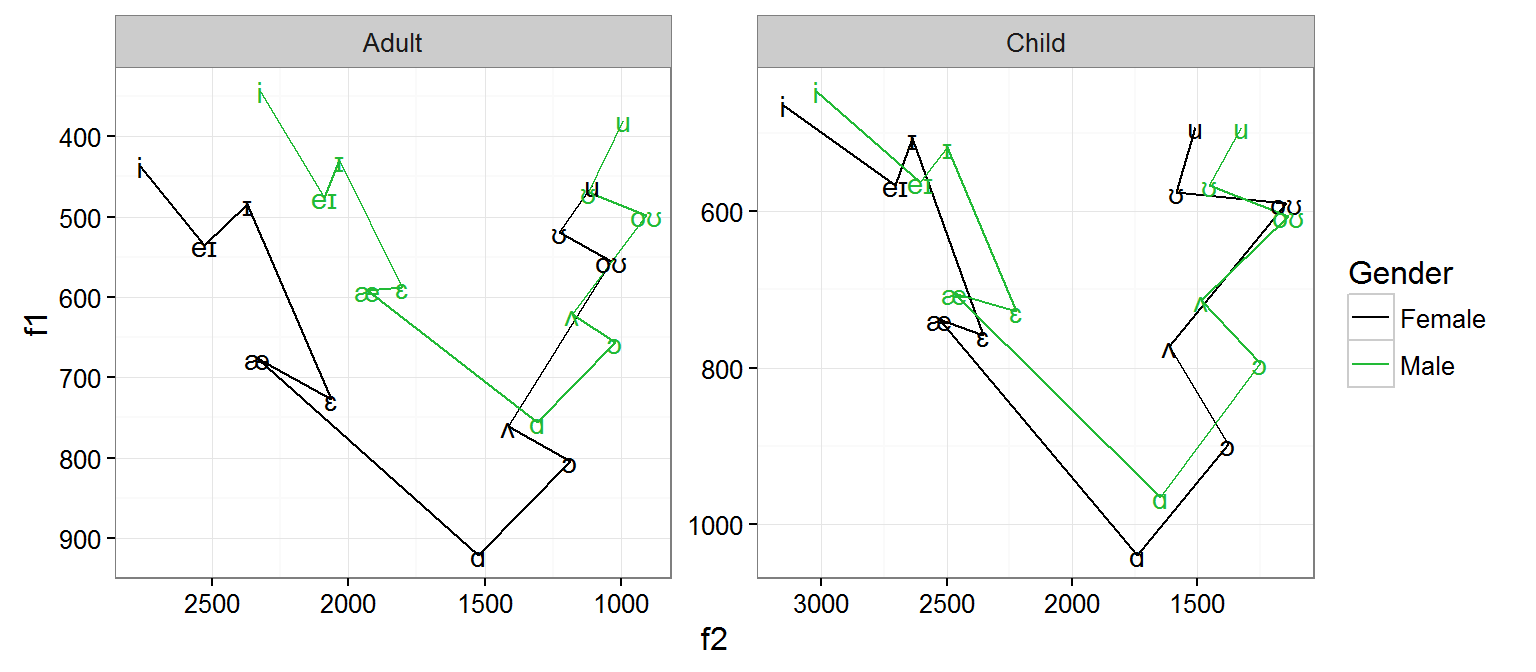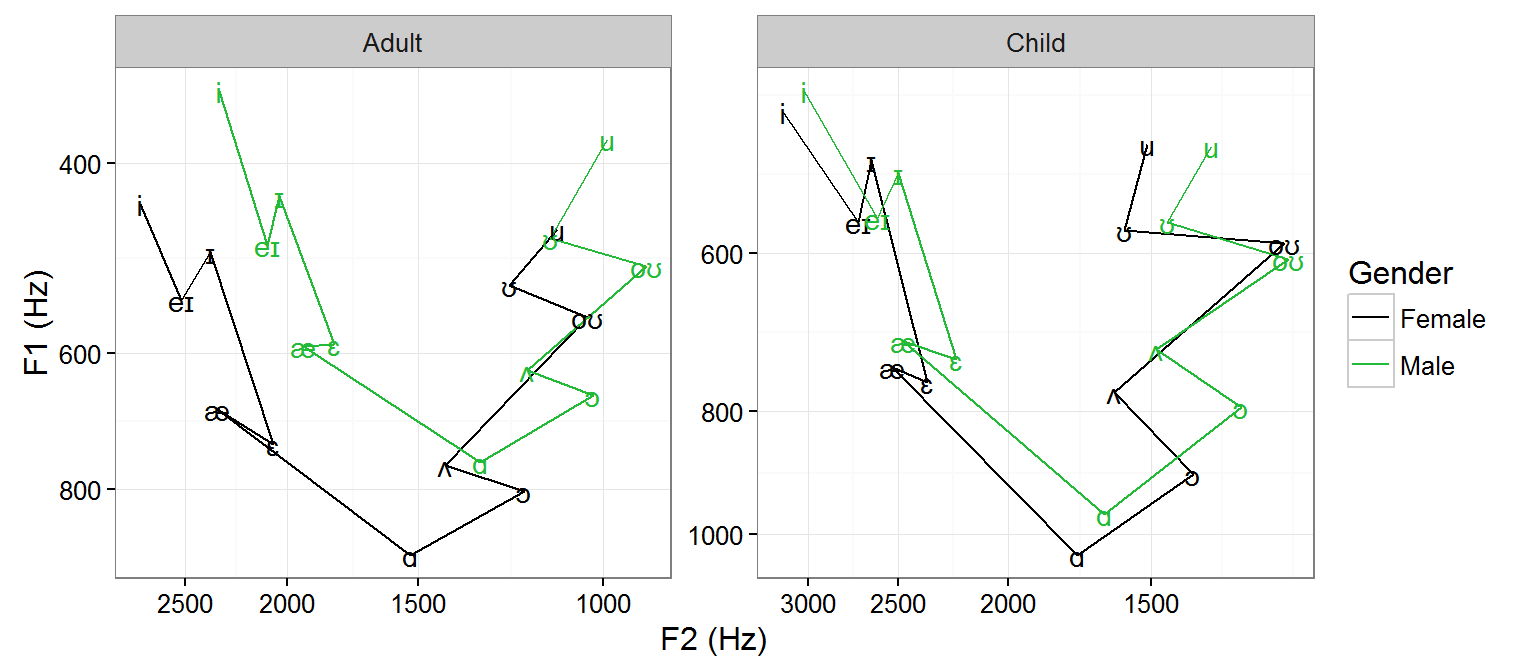## Plotting vowels using the Hillenbrand et al. (1995) data set

### This is a tutorial on how to visualize vowel formant measurements using the Hillenbrand et al. (1995) data base. The script assumes that you have already created the R data objects that were produced using the “Cleaning and formatting vowel data” tutorial. If you haven’t downloaded that, you can just download the objects here.

All data objects in this tutorial come from that .RData file and can be examined thoroughly by loading them or viewing the first tutorial.

### First Load the required packages

``````library("ggplot2")
library("dplyr")
library("scales")
library("animation")``````

Note that the animation function requires installation of ImageMagick.

### Call up the data:

First guide R to the directory where the R objects live on your computer (i.e. where you put it from the first tutorial, or where you just downloaded the data using the link above).

``````folder_with_saved_objects <- "C:\\Enter\\Your\\Folder\\Path\\Here"
setwd(folder_with_saved_objects)

## Plot steady-state formant values from ALL tokens

Use the `data.wide.ss` object, as it contains formant values for each of the vowels grouped by talker gender and age. The data is in “wide” format because each of the formnt values is coded as a different variable (as opposed to being a single column, whose formant number is coded in an adjacent column). Wide-format data is required when setting different formants (e.g. F1 nd F2) to separate axes.
Note how for each talker group, we let each axis limits adjust to the range of data for that group using `scales="free"`.

``````px_all_pts <- ggplot(data.wide.ss)+
aes(x=f2, y=f1,
label=Vowel.IPA,
color=Vowel.ordered)+
scale_x_reverse(name="F2 (Hz)")+scale_y_reverse(name="F1 (Hz)")+
geom_text()+
theme_bw()+
theme(legend.position="none")+
facet_wrap(~ Age + Gender, scales="free")
px_all_pts``````## Plot simple means for each vowel

While it is easy to produce a `mean` of the y-axis value inline in the ggplot code (e.g. with `stat_summary()`), it’s not clear how to do that for the y-axis and the x-axis at the same time. And yet we want to keep the same plot setup that we just produced with the previous lines of code. So what we need to do is simply substitute a different data frame to the same plot using `%+%` (subbing the `data.wide.ss.sum` data frame in place of the one without “.sum”) and set a new label aesthetic to remove the redundant color info.

``````px_vowel_means_4_panel <- px_all_pts %+%
data.wide.ss.sum +
aes(label=Vowel.IPA, color=NULL)+
guides(color="none")
px_vowel_means_4_panel``````## Plot Vowel space

Add a line path to define vowel space (this is why we declared a vowel order in the first script).

This code takes a data frame and first filters out the ‘er’ vowel because it’s a bit of an odd case. Then, within each group defined by Age and Gender, it arranges the data frame so that the rows are ordered by the vowel order established in the first script (it is essentially a counter-clockwise walk around the vowel space) and sends it into the `ggplot()` function.

``````px_v_space_by_gender <- data.wide.ss.sum %>%
dplyr::filter(Vowel!="er") %>%
group_by(Age, Gender) %>%
arrange(as.numeric(Vowel.order.num)) %>%
ungroup %>%
ggplot(., aes(x=f2, y=f1, color=Gender))+
geom_path()+
geom_text(aes(label=Vowel.IPA), show.legend=FALSE)+
scale_x_reverse() + scale_y_reverse()+
theme_bw()+
scale_color_manual(values = c("Female" = "black",
"Male" = "#22BA36"))+
facet_wrap( ~ Age, scales="free")
px_v_space_by_gender``````## Convert axes to log scales

Here, a specialized funciton is needed to create a log scale in reverse:

``````reverselog_trans <- function(base = exp(1)) {
trans <- function(x) -log(x, base)
inv <- function(x) base^(-x)
trans_new(paste0("reverselog-", format(base)), trans, inv,
log_breaks(base = base),
domain = c(1e-100, Inf))
}``````

This can be used simply as follows:

``````# p = a plot that you have already created
p_reverse_log <- p + scale_x_continuous(trans=reverselog_trans(10))``````

Now let’s add it to our plot:

``````px_v_space_by_gender.log <- px_v_space_by_gender +
scale_x_continuous(name="F2 (Hz)",
trans=reverselog_trans(10),
breaks=seq(1000,3000,500))+
scale_y_continuous(name="F1 (Hz)",
trans=reverselog_trans(10),
breaks=seq(400,1000,200))``````
``````## Scale for 'x' is already present. Adding another scale for 'x', which
## will replace the existing scale.
## Scale for 'y' is already present. Adding another scale for 'y', which
## will replace the existing scale.``````
``px_v_space_by_gender.log``Now the data are treated in a way that more closely resembles the auditory transformation of the vowel space. There are other clever ways of doing this (using the Bark scale, ERB, or the Greenwood function).

Note fo explanatory purposes that the axis labels are not labeled with “log Hz” - they are labeled with linear Hz, and the spacing is simply transformed. Log-Hz values would take values between roughly 5.7 and 7.9 (for natural log) or between 2.5 and 3.4 (for base-10 log).

### Plot both adults & children on the same plot

… and distinguish them by line type

``````px_v_space_by_gender_age <- px_v_space_by_gender+
aes(linetype=Age) +
facet_null()
px_v_space_by_gender_age``````